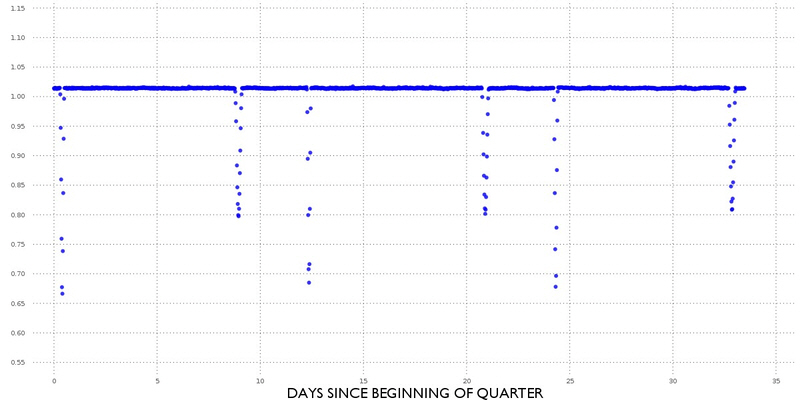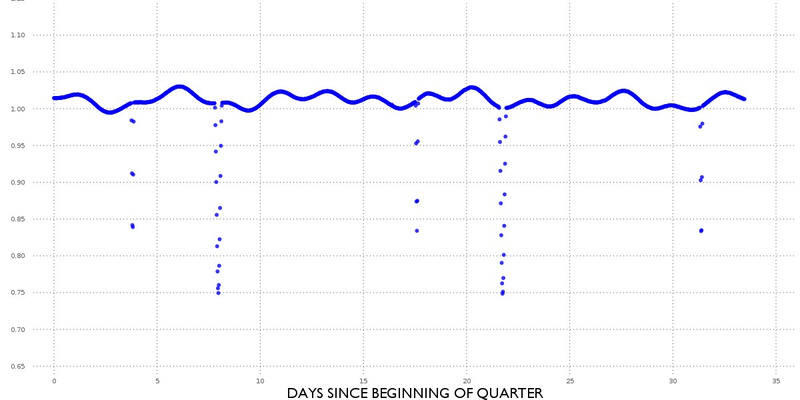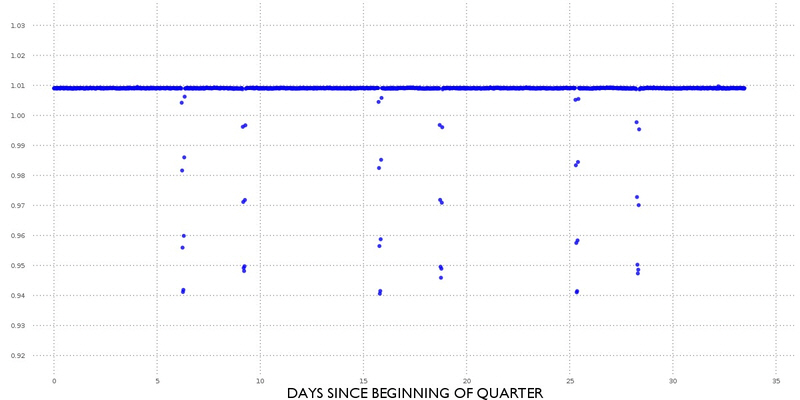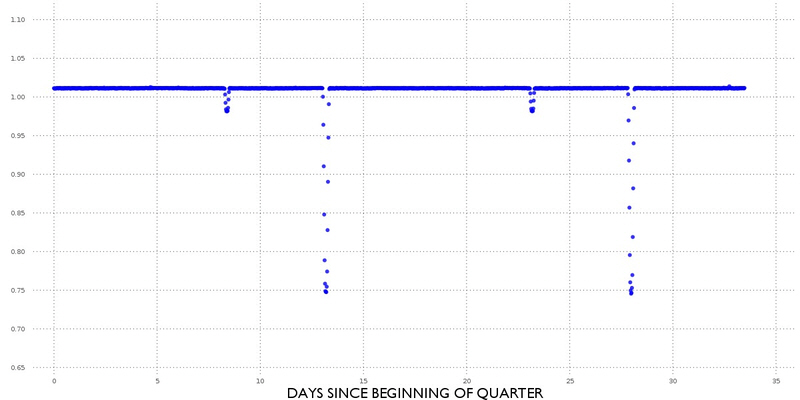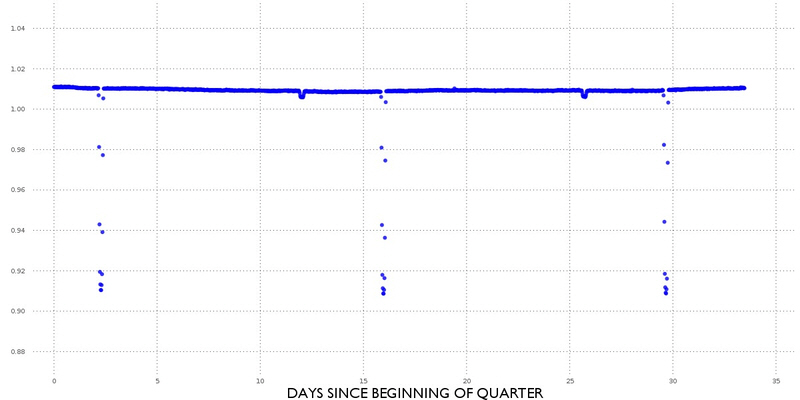Kepler - eccentric binary stars

This site is currently under construction. Things will change regularly.

The orbital eccentricity of an astronomical body is the amount by which its orbit deviates from a perfect circle, where 0 is perfectly circular, and 1.0 is a parabola, and no longer a closed orbit.

There is a positive correlation between the period of revolution of a binary star and the eccentricity of its orbit. This means that on average the longer the period of a binary star system the greater the orbital eccentricity.

The Eclipsing Binary Simulator from the University of Nebraska Lincoln can be used to demonstate the light curves than be found for binary star systems of different eccentricities.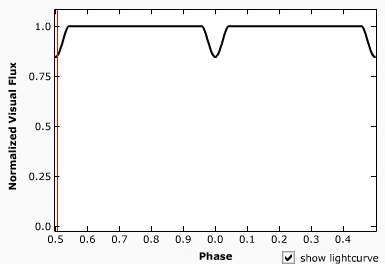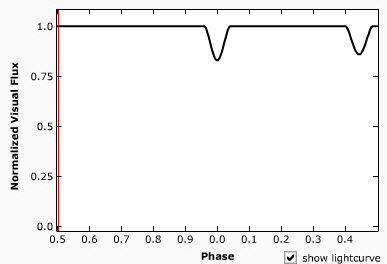Eccentricity = 0 Eccentricity = 0.1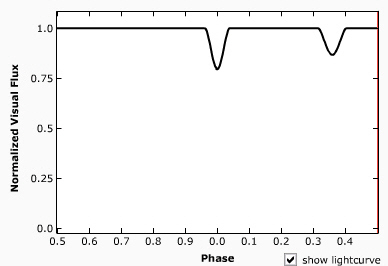Eccentricity = 0.25 Eccentricity = 0.5

EXAMPLES FROM THE KEPLER RESULTS